# Calculating the number of days between two dates

You are here:
• Calculating the number of days between two dates

This worksheet shows how to calculate the number of days between two dates. The example used is for a questionnaire asking about a participant’s vacation where the period of time the participant spent on vacation is calculated. Additionally, there is an analysis requirement to determine the average length of vacation by age of respondent and to group the vacations into length of vacation bands.

The questionnaire needs to ask for the date when the participants went on vacation, the date they returned and their age in order to calculate the analysis requirements.

### Step 1: Create a variable for the number of days away

1. Enter two open ended, date response questions, one for the vacation departure date and one for the vacation return date. Then create Q3 using a multiple-choice, single response question to capture respondent’s ages. The questions are shown here.
1. From the Variables window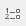click New Variable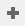to create the variable that will contain the formula to work out the number of days between the two dates
1. Enter the following information:
• Name: DaysAway
• Label: Days Away on Vacation
• Type: Derived
• Response: Quantity
• Text: Days Away on Vacation
2. In the Values column, in the same row as Valid, type Q2-Q1. This will calculate the number of days between holiday departure date and holiday return date.

### Step 2: Create a table showing the average length of stay by age

1. Click on Analysis Table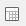. This opens the Analysis Definition dialog.
1. In Analysis, enter Q3 What is your age?
2. In the Calculate dropdown select Means and Significances and in the box to the right enter the derived variable, DaysAway.
3. Click OK to show results.

### Step 3: Grouping the number of days on vacation into bands

This will categorise the data so that a table or chart can be produced.

1. From the Variables windowclick New Variableto create the variable to show the amount of days from the departure date to the return date.
2. Enter the following information:
• Name: Length
• Label: Length of Vacation
• Type: Derived
• Response: Single
3. In the Code list, click the Label field for Code 1 and enter 1 to 3 days. Press Tab on the keyboard, to move to the Values column. In this column Snap needs to know what numbers are to be displayed in this answer, enter 1 to 3. Follow the same procedure to create categories for 4 to 6 days, 7 to 10 days and over 10 days.
1. Click on Toggle Definitions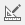and change Calculation to DaysAway.
2. Click Save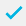to save.
3. Click on Analysis Tableto show the Analysis Definition window.
4. In Analysis enter Length. Click OK to show results.

If there is a topic you would like a worksheet on, email to snapideas@snapsurveys.com